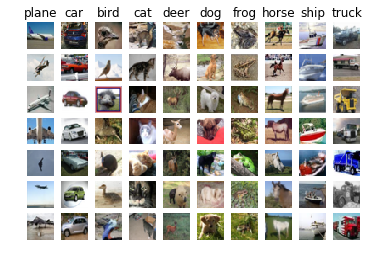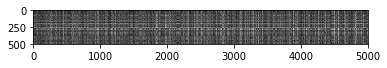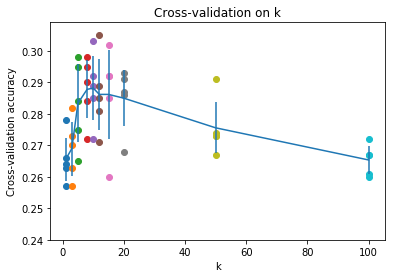# [cs231n] assignment1 KNN部分

## 1.前言

• 图像处理的数据集CIFRAR-10
• kNN的实现
• 交叉验证
• 用numpy实现向量化

## 2.具体实现部分

kNN算法比较直观，对于每一个测试集的数据，去找和它相邻的最近的k个训练集的数据，然后把它们对应的label进行投票，投票最多的那个就是这个测试集的输出。直接贴上代码，上面一般都附上了注释：

### k-Nearest Neighbor (kNN) exercise

Complete and hand in this completed worksheet (including its outputs and any supporting code outside of the worksheet) with your assignment submission. For more details see the assignments page on the course website.

The kNN classifier consists of two stages:

• During training, the classifier takes the training data and simply remembers it
• During testing, kNN classifies every test image by comparing to all training images and transfering the labels of the k most similar training examples
• The value of k is cross-validated

In this exercise you will implement these steps and understand the basic Image Classification pipeline, cross-validation, and gain proficiency in writing efficient, vectorized code.We would now like to classify the test data with the kNN classifier. Recall that we can break down this process into two steps:

1. First we must compute the distances between all test examples and all train examples.
2. Given these distances, for each test example we find the k nearest examples and have them vote for the label

Lets begin with computing the distance matrix between all training and test examples. For example, if there are Ntr training examples and Nte test examples, this stage should result in a Nte x Ntr matrix where each element (i,j) is the distance between the i-th test and j-th train example.

First, open cs231n/classifiers/k_nearest_neighbor.py and implement the function compute_distances_two_loops that uses a (very inefficient) double loop over all pairs of (test, train) examples and computes the distance matrix one element at a time.Inline Question #1: Notice the structured patterns in the distance matrix, where some rows or columns are visible brighter. (Note that with the default color scheme black indicates low distances while white indicates high distances.)

• What in the data is the cause behind the distinctly bright rows?
• What causes the columns?

You should expect to see approximately 27% accuracy. Now lets try out a larger k, say k = 5:

You should expect to see a slightly better performance than with k = 1.

Inline Question 2
We can also other distance metrics such as L1 distance.
The performance of a Nearest Neighbor classifier that uses L1 distance will not change if (Select all that apply.):
1. The data is preprocessed by subtracting the mean.
2. The data is preprocessed by subtracting the mean and dividing by the standard deviation.
3. The coordinate axes for the data are rotated.
4. None of the above.

### Cross-validation

We have implemented the k-Nearest Neighbor classifier but we set the value k = 5 arbitrarily. We will now determine the best value of this hyperparameter with cross-validation.Inline Question 3
Which of the following statements about $k$-Nearest Neighbor ($k$-NN) are true in a classification setting, and for all $k$? Select all that apply.
1. The training error of a 1-NN will always be better than that of 5-NN.
2. The test error of a 1-NN will always be better than that of a 5-NN.
3. The decision boundary of the k-NN classifier is linear.
4. The time needed to classify a test example with the k-NN classifier grows with the size of the training set.
5. None of the above.

## 总结## Ref

MATLAB计算矩阵间的欧式距离（不用循环！）